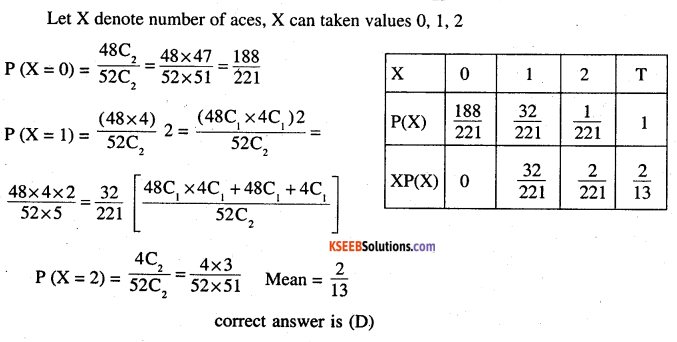# 2nd PUC Maths Question Bank Chapter 13 Probability Ex 13.4

Students can Download Maths Chapter 13 Probability Ex 13.4 Questions and Answers, Notes Pdf, 2nd PUC Maths Question Bank with Answers helps you to revise the complete Karnataka State Board Syllabus and score more marks in your examinations.

## Karnataka 2nd PUC Maths Question Bank Chapter 13 Probability Ex 13.4

### 2nd PUC Maths Probability NCERT Text Book Questions and Answers Ex 13.4

Question 1.
State which of the following are not the probability distributions of a random variable. Give reasons for your answer.
(i)

 x 0 1 2 P(X) 0.4 0.4 0.2

 X 0 1 2 T P(X) 4 4 2 1

(ii)

 X 0 1 2 3 4 P(X) 0.1 0.5 0.2 -0.1 0.3

 X 0 1 2 3 T P(X) 0.1 0.5 0.2 -0.1 1

It is not P.d as P(X) < 0 when x = 3(iii)

 Y -1 0 1 P(Y) 0.6 0.1 0.2

 Y -1 0 1 T P(Y) 0.6 0.1 0.2 .9

It is not P.d as ΣP(X) = .9 ≠1

(iv)

 Z 3 3 1 0 -1 P(Z) 0.3 0.2 0.4 0.1 0.05

 Z 3 3 1 0 T P(Z) 0.3 0.2 0.4 0.1 1.05

It is not a P.d as ΣP(X) = 1.05 > 1

Question 2.
An urn contains 5 red and 2 black balls.Two balls are randomly drawn. Let X represent the number of black balls. What are the possible values of X ? Is X a random variable ?
X can take the values 0, 1, 2 It is a Random variable because the experiment is a random experiment.

Question 3.
Let X represent the difference between the number of heads and the number of tails obtained when a coin is tossed 6 times. What are possible values of X?
When m = 6, n = 0  ⇒ |m-n| = 6
m = 5,n = 1                  |m-n| = 4
m = 4, n = 2                 |m-nl=2
m = 3,n = 3                  |m-n| = 0
m = 2, n = 4                 |m-n|=2
m = 1,n = 5                  |m-n| = 4
m = 0, n = 6                 |m-n|=6
∴ hence X can take values 0, 2, 4, 6.

Question 4.
Find the probability distribution of
(i) number of heads in two tosses of a coin.
(ii) number of tails in the simultaneous tosses of three coins.
(iii) number of heads in four tosses of a coin.
(i) When a coin is tossed twice, the number of heads may be 0, 1,2
Sample space {HH, HT, TH, TT}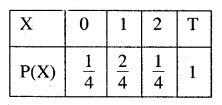(ii) When a coin is tossed thrice, the number of heads are 0, 1, 2, 3
Sample space {HHH, HHT, HTH, HTT, ITT, TTH, THT, THH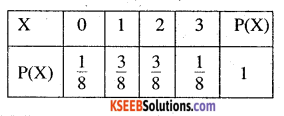(iii) When coin is tossed four times, the number of heads be 0, 1, 2, 3,4 Sample space {HHHH, HHHT, HHTH, HHTT, HTHT, HTHH, HTTH, TTTT, TTTH, HTTT, TTHT, TTHH, THTH, THTT, THHT, THHH]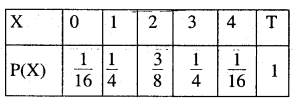Question 5.
Find the probability distribution of the number of successes in two tosses of a die, where a success is defined as
(i) number greater than 4
(ii) six appears on at least one die
Ans:
(i) Let the random variable be X no of successes then X can take the value 0,1,2.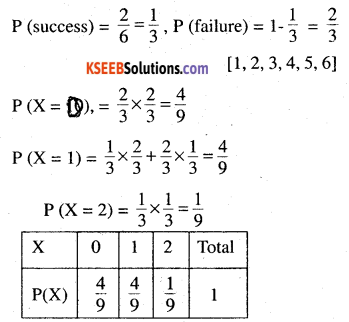(ii) Let X be random variable, then X can take values
O (no sixes) & 1 (at least 1 six)
P (x = 0) = P (no six)
= [1-P (getting a six)] x [1 – P (getting a six on single throw]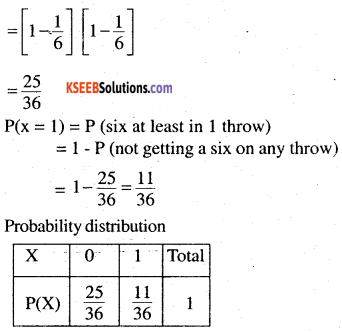Question 6.
From a lot of 30 bulbs which include 6 defectives, a sample of 4 bulbs is drawn at random with’ replacement. Find the probability distribution of the number of defective bulbs.
Let X denote the no. of defective bulbs P denote the probability of obtaining defective bulb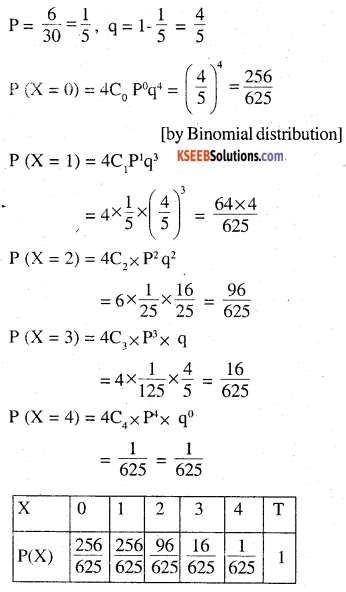Question 7.
A coin is biased so that the head is 3 times as likely to occur as tail. If the coin is tossed twice, find the probability distribution of number of tails.
Let X denote the number of tails in two tosses of a coin
∴ X can assain the values 0, 1,2
Let P be the probability getting head, q the tail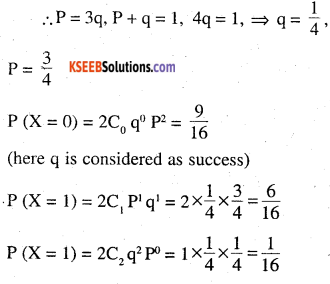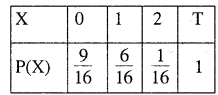Question 8.
A random variable X has the following probability distribution:

 X 0 1 2 3 4 5 6 7 P(X) 0 k 2k 2k 3k k2 2k2 7k2+k

Determination
(i) K
(ii) P(X<3)
(iii) P(X>6)
(iv) P(0<X<3)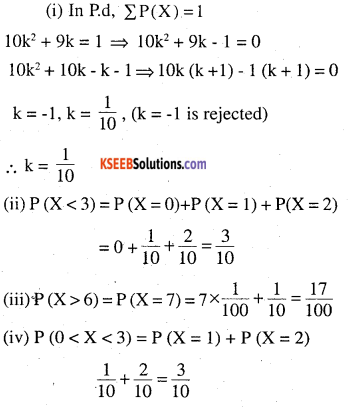Question 9.
The random variable X has a probability distribution P(X) of the following form, where k is some number:
$$P(X)=\left\{\begin{array}{ll}{k,} & {\text { if } \quad x=0} \\{2 k,} & {\text { if } x=1} \\{3 k,} & {\text { if } x=2} \\{0,} & {\text { otherwise }}\end{array}\right.$$
(a) Determine the value of k
(b) Find P (X < 2), P (X≤2), P (X≥2)
(a) In P.d. Σ XP(X)= 1
6k = 1 ⇒ $$k=\frac{1}{6}$$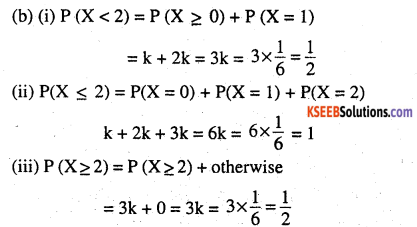Question 10.
Find the mean number of heads in three tosses of a fair coin.
If three coins are tossed, Let X denote the number of heads.
X can take the values 0, 1, 2, 3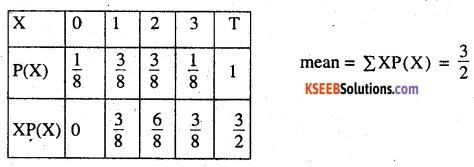Question 11.
Two dice are thrown simultaneously. If X denotes the number of sixes, find the expectation of X.
Let X denote the number of sires. Then X can take values 0,1,2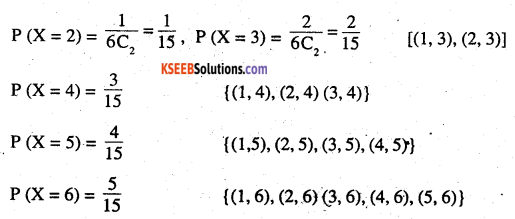Question 12.
Two numbers are selected at random (without replacement) from the first six positive integers. Let X denote the larger of the two numbers obtained. Find E(X).
Ans:
X can take the values 2, 3, 4, 5, 6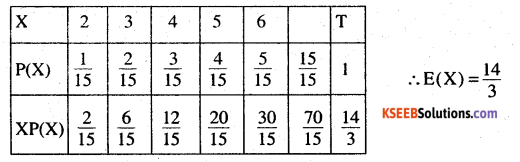Question 13.
Let X denote the sum of the numbers obtained when two fair dice are rolled. Find the variance and standard deviation of X.
Ans:
Let X denote the sum of the numbers obtained
∴  X can take the values 2, 3,4, 5,6,7, 8,9, 10, 11, 12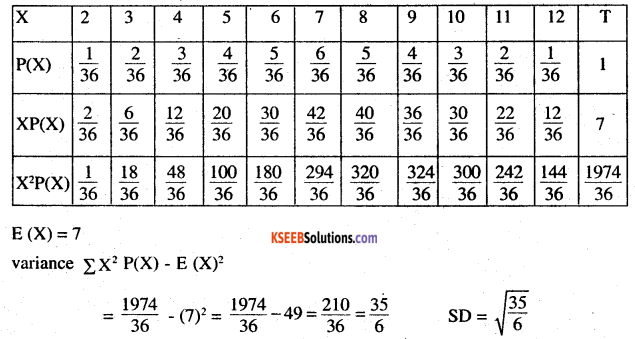Question 14.
A class has 15 students whose ages are 14, 17, 15, 14, 21, 17, 19, 20, 16, 18, 20, 17, 16, 19 and 20 years. One student is selected in such a manner that each has the same chance of being chosen and the age X of the selected student is recorded. What is the probability distribution of the random variable X? Find mean, variance and standard deviation of X.
Ans:
Let X denote the age of the students.
Then X can take the values 14,15,16,17, 18,19,20, 21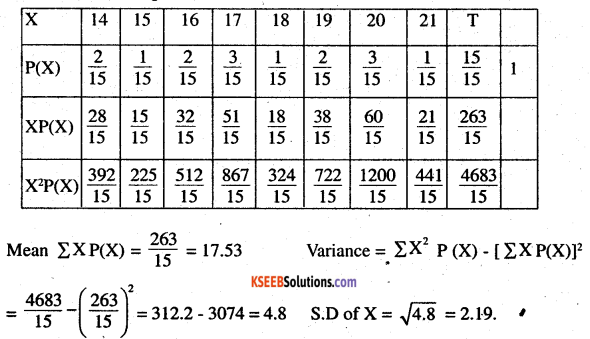Question 15.
In a meeting, 70% of the members favour and 30% oppose a certain proposal. A member is selected at random and we take X = 0 if he opposed, and X = 1 if he is in favour. Find E(X) and Var (X).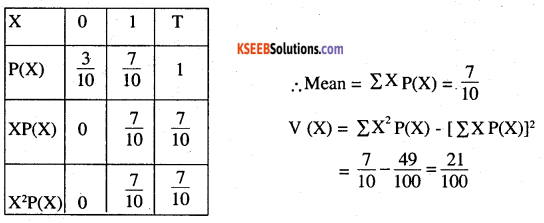Choose the correct answer in each of the following:

Question 16.
The mean of the numbers obtained on throwing a die having written 1 on three faces, 2 on two faces and 5 on one face is
(A) 1
(B) 2
(C) 5
(D) 1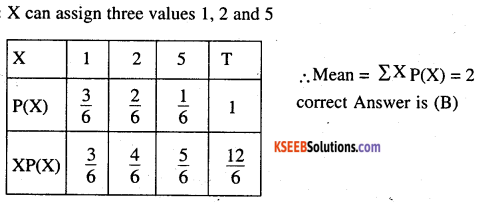(A) $$\frac{37}{221}$$
(B) $$\frac{5}{13}$$
(C) $$\frac{1}{13}$$
(D) $$\frac{2}{13}$$